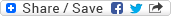## Wednesday, May 28, 2008

### Probability of seeing a car in 5 minutes - Puzzle

Puzzle: If the probability of observing a car on a highway in 20 minutes time is 609/625 then what is the probability of observing a car in 5 minutes time on the same highway (considering all the factors involved to be uniform)?

Solution: Probability of seeing a car in 20 minutes = 609/625
=> Probability of not seeing a car in 20 minutes = 1 - 609/625 = 16/625
=> (Probability of not seeing a car in 5 minutes)^4 = 16/625
=> Probability of not seeing a car in 5 minutes = (16/625)^(1/4)
=> Probability of not seeing a car in 5 minutes = 2/5

Hence, the Probability of seeing a car in 5 minutes = 1 - 2/5 = 3/5